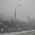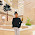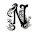VHDL coding tips and tricks: A VHDL function for division two unsigned numbers

## Wednesday, March 17, 2010

### A VHDL function for division two unsigned numbers

I have written a function for division of variables in VHDL.The function is based on "Restoring Division algorithm".The function takes two unsigned numbers(dividend and divisor) of the same size and returns the quotient,which is also of unsigned type with the same size.The function is a generalized one and can be used for any size of inputs.

function  divide  (a : UNSIGNED; b : UNSIGNED) return UNSIGNED is
variable a1 : unsigned(a'length-1 downto 0):=a;
variable b1 : unsigned(b'length-1 downto 0):=b;
variable p1 : unsigned(b'length downto 0):= (others => '0');
variable i : integer:=0;

begin
for i in 0 to b'length-1 loop
p1(b'length-1 downto 1) := p1(b'length-2 downto 0);
p1(0) := a1(a'length-1);
a1(a'length-1 downto 1) := a1(a'length-2 downto 0);
p1 := p1-b1;
if(p1(b'length-1) ='1') then
a1(0) :='0';
p1 := p1+b1;
else
a1(0) :='1';
end if;
end loop;
return a1;

end divide;

The function can be used as follows in your main module:
--An example of how to use the function.
signal a : unsigned(7 downto 0) :="10011100";
signal b : unsigned(7 downto 0) :="00001010";
signal c : unsigned(7 downto 0) :=(others => '0');
c <= divide ( a , b );  --function is "called" here.

For using the function you have to copy the code snippet between the green lines and put it in a package.The libraries I have used are given below:

library IEEE;
use IEEE.std_logic_1164.all;
use ieee.numeric_std.all;      -- for UNSIGNED

If you don't know about how to include functions in packages then you can learn it here.

Note :- This function is synthesizable.The code doesn't work for negative numbers.

#### 30 comments:

1.is this function working for std_logic type???

2.is it possible to do division or modulus operation in xilinx ise without using any function or any user own logic codes???

3.@rourab : Why do you want to divide std_logic types. You can then use some other simple code to get the result.
The division operator available in vhdl has some limitations.But in Xilinx, you can use the "divider generator" IP core for division if you want.

4.I have used divider operator('/') in ISE tool in vhdl code. the test bench is fine, but when im going to implement it on fpga board its giving error. it is saying the divider must be power of two . why ?

5.@rourab : it happens. Use this function for division.

6.thank you for reply.
actually i want modulus(%) operation. I have already implemented your function on hardware(vertex 5), i just add a extra line
"m1:=a-(a1*b);" where m1 hold the modulus value.
but particularly this line (a subtraction and multiplication) take extra resources on FPGA board for large data width input. So i want to avoid this line. I am not going through in your algorithm. Using this algorithm is there any way where i can find modulus value directly

7.@rourab: there is a "mod" operator in vhdl for doing the modulo operation on integers. You can use that.

8.I have tried all the things, every thing is fine in testbench, but in case of implemantation purpose these operators like mod, divider are not working. you can try it .. could you modify your algorithm?

9.@rourab :I think it should be working. The above code does what it is supposed to do.

For your purpose I suggest you the "mod" operator available in numeric_std library. All the source and destination operands should be unsigned.

function "mod" (L, R: UNSIGNED) return UNSIGNED;

Google for "mod" operator and you will find lot of examples.

10.library IEEE;
use IEEE.STD_LOGIC_1164.ALL;
use IEEE.STD_LOGIC_ARITH.ALL;
use IEEE.STD_LOGIC_UNSIGNED.ALL;
use ieee.numeric_std.all;

---- Uncomment the following library declaration if instantiating
---- any Xilinx primitives in this code.
--library UNISIM;
--use UNISIM.VComponents.all;
entity motor is --constrained in ucf file
Generic ( n : positive := 4);
Port (
clk : in std_logic; --spartan 3 e
input_data : in std_logic_vector((n-1) downto 0);
decrypted_data : inout std_logic_vector((n-1) downto 0)
);
end motor;

architecture Behavioral of motor is

signal base : unsigned ((n-1) downto 0):="1010";
signal temp : unsigned ((n-1) downto 0);
begin

temp<= unsigned(input_data) mod base;
decrypted_data<=std_logic_vector(temp);
end Behavioral;

I have tried this code
the error is
"mod can not have such operands in this context"

11.@rourab : Use only numeric_std. And dont use std_logic_vector.
And write a simple code to test the use of mod. You are complicating it with so many type conversions.

12.Now i have used
--------------------------------
library IEEE;
use IEEE.STD_LOGIC_1164.ALL;
use ieee.numeric_std.all;
--------------------------------
--and ports are
---------------------------
Port (
--clk : in unsigned; --spartan 3 e
input_data : in unsigned((n-1) downto 0);
decrypted_data : inout unsigned((n-1) downto 0)
);
-----------------------------------
--a signal
---------------------------------
signal base : unsigned ((n-1) downto 0):="1010";
---------------------------------------------
and i have written
decrypted_data<= input_data mod base;
---------------------------------------
testbench is fine but when im trying to implement it, its saying
"Operator must have constant modulo operand."
when i declare the base signal as constant
its saying
"The modulo should be a power of 2"
what should i do??

13.@rourab : try "rem" instead of mod and see what happens.
which version of ISE are you using?

14.Im using ise 11.1
i have tried rem,
its saying
"Operator must have constant operands or first operand must be power of 2"

15.I think your tool doesnt support the mod operator fully.

I am not sure about this, but can you use the above code by making the following change:
return a1; REPLACE IT WITH return p1;

If this also doesnt work then contact me through the "contact me" page.

16.this works fine vipin.
Vipin now i have have problem.
i want to use this function as a separate block.
i habe written following code.
entity motor is --constrained in ucf file
Generic ( n : positive := 3);
Port (
clk : in std_logic; --spartan 3 e

dividend : in std_logic_vector((n-1) downto 0);
divisor : in std_logic_vector((n-1) downto 0);

remainder : out std_logic_vector((n-1) downto 0)
);
end motor;

architecture Behavioral of motor is

signal a : unsigned((n-1) downto 0):= (others => '0');
signal b : unsigned((n-1) downto 0):= (others => '0');

--================================================================
signal p1_out : unsigned(divisor'length downto 0):= (others => '0');
signal a1 : unsigned(dividend'length-1 downto 0):=unsigned(dividend);
signal b1 : unsigned(divisor'length-1 downto 0):=unsigned(divisor);
signal p1 : unsigned(divisor'length downto 0):= (others => '0');
begin

main: process(clk )

variable i : integer:=0;

begin

if(rising_edge(clk)) then

for i in 0 to divisor'length-1 loop
p1(divisor'length-1 downto 1) <= p1(divisor'length-2 downto 0);
p1(0) <= a1(dividend'length-1);
a1(dividend'length-1 downto 1) <= a1(dividend'length-2 downto 0);
p1 <= p1-b1;
if(p1(divisor'length-1) ='1') then
a1(0) <='0';
p1 <= p1+b1;
else
a1(0) <='1';
end if;

end loop;

end if;
p1_out<=p1;

end process main;
remainder<=std_logic_vector(p1_out((divisor'length-1) downto 0));

end Behavioral;

===========================================
i replace all of the variable by signal.
but i didnt get the desired result
p1, a1 and b1 did not get the any value. why this is happening??

17.Hi vipin,

I tried this function in my Design. Unfortunately, it doesn't give the correct value..

I have 2 questions..

1. variable p1 : unsigned(b'length downto 0):= (others => '0');

Is the length declaration correct here?

2. if(p1(b'length-1) ='1') then
What this means? The Restoring algorithm has no condition like this..

Thank you

18.1.The varibale size pi is absolutely fine.
2. If the width of B input is 'n' then
"if(p1(b'length-1) ='1')" it check the (n-1) for logic '1'

19.@rourab...

Thanks for the reply. It works good now..

But, the function c <= divide ( a , b ), works only when a and b are fixed values.

I tried generating random no.s of a and b of same width, the function doesn't provide the correct output...

20.@swami:but i have implemented this code successfully with random no. in that case i would prefer to check ur code,
@vipin: could u help me about my problem?

21.@rourab : are you having problems with changing variables to signals? this is because variables gets updated immediately and signals after a delta delay.
to make it work with signals you can do one thing. Re-write the code in the form of a state machine.Each assignment or calculation happens in one clock cycle or in one state of the state machine.

22.@vipin
Obviously I will implement the design using state machine. But here i have very basic qusetion about harware designig. I may be sounded like a fool. If i write this design using state machine it will take sevaral clocks,design will be slow. Here in a single clock the design will execute less no. of operations compare to "without state machine designing"(i,e in fuctions). In that case what will be changed in resouces utilization? could FPGA reuse the slices which were used in previous clock cycle?

23.@rourab : state machines will lead to less throughput and but less resources.

24.@all: help to me creat arithmetic paperlined block and this block use division 3 numbers 8 bit. Thank you very much.

25.@Vipin: How to get the reminder of the fractional value.

26.Thanks guys, you helped me so much, you have super blog :)

27.I tried ur code..but it was showing error,i could not arrange codes properly may be,can tell me the steps to arrange the codes?

28.guys does any one can post their suggeston
....where 256x256 array values need to implented over an fgpa

1.You can store image values in Text file and then you can use it over FPGA.

29.Sir I have tried to implement your above given code, but it is not working.Will You please help me for the same.Thank you in advace.